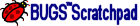Chapter 3. Elements of an Aquifer Simulation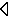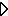## 3.3 Reactions

Reactions are a very important part of a aquifer system. They manipulate values of parameters as a function of time and other parameters. (Mobile parameters are also manipulated relative to space and time as a function of their advection-dispersion properties.) There are two types of reactions, equilibrium and kinetic.

Equilibrium reactions are instantaneous while kinetic reactions are rate limited. Each reaction requires two types of data; rate data (for kinetic), rule data (for equilibrium) and Stoichiometry data. For example consider the equilibrium reaction: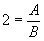(3.1)

Equation (3.1) states the ratio of parameter A to Parameter B must always be two. Setting this rule alone is insufficient. It is necessary to tell BUGS how these two parameters relate to each other so the adjustment can take place (and the mass balance preserved). An example of the stoichiometry corresponding to equation (3.2) is: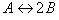(3.2)

With the stoichiometry relationship, BUGS SCRATCHPAD will know how much of A appears when B disappears, or the opposite. This is similar for kinetic reactions. Consider the Reaction: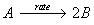(3.3)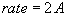(3.4)

Equation (3.3) proceeds forward at the rate specified by equation (3.4).

BUGS SCRATCHPAD recognizes many different types of Equilibrium and Kinetic Reactions, including: Equilibrium, Ordered, Monod, Competitive Inhibition, Linear Isotherm, Fedulich Isotherm, and Langmuir Isotherm. Also, a rule curve reaction is included for special cases. Specific information on each reaction can be found later in this document.

It should be noted that BUGS SCRATCHPAD does not use any particular set of units in its simulation. Units are assumed to be "consistent". The user has to make the conversions from mass to moles if necessary.

Last Updated October 1, 2001